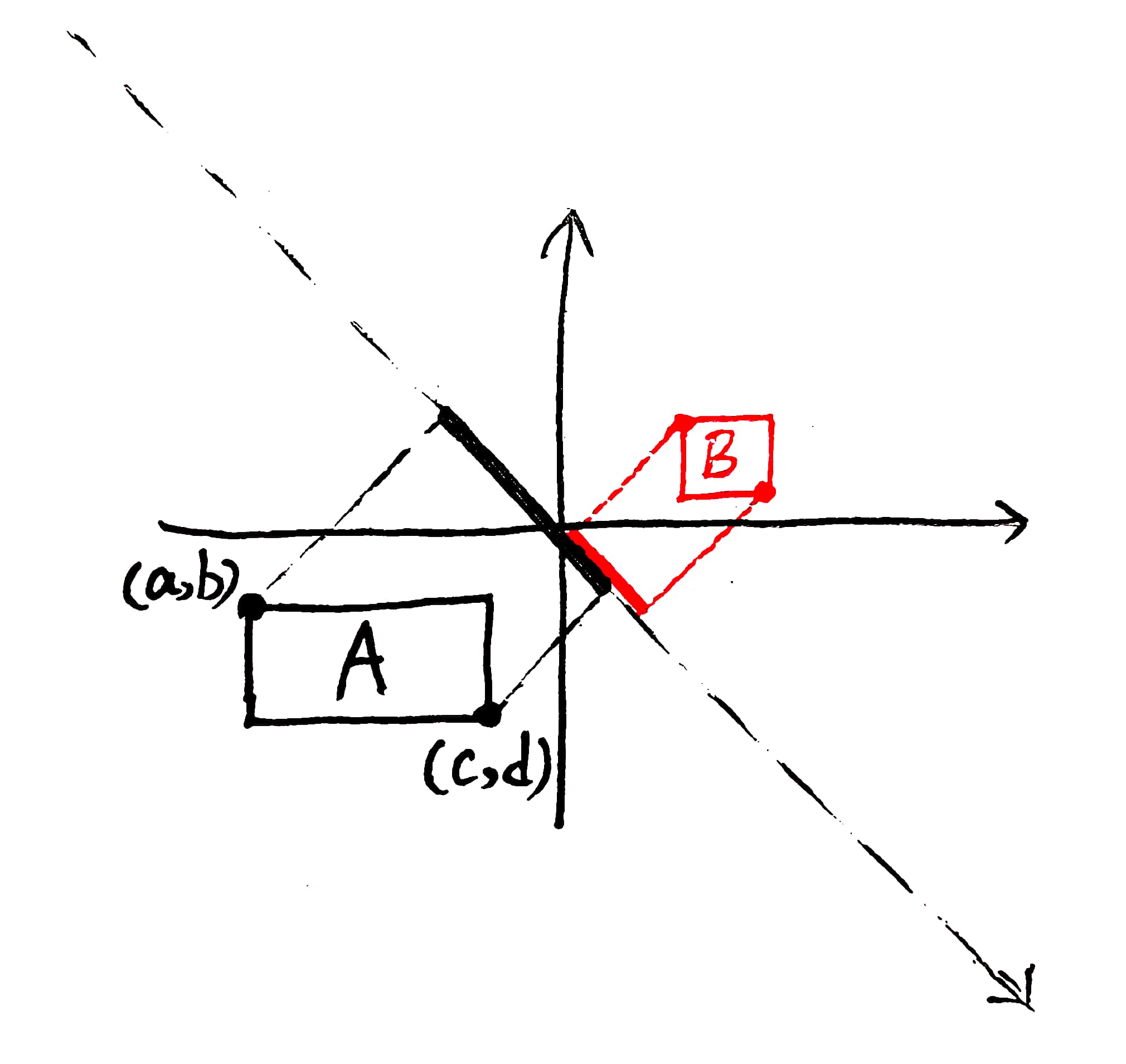# 2020牛客寒假算法基础集训营6

2020-02-18 增加H题题解

# A. 配对

## 题目描述

$1\leq K\leq N\leq100,000$ 输入的所有数字不超过 $10^8$

# B. 图

## 题目描述

$(1\leq N\leq1,000,000)$

## 输入描述

（点从 $1$ 开始标号）

# C. 汉诺塔

## 题解

待补充


# D. 重排列

## 输入描述

$1\leq N\leq100,000$ 且 $0\leq A_i,B_i\leq10^9$

## 题解

$$Answer =\prod_{i=1}^{N}(A\,\text{序列中不大于}\,B_i\,\text{的项数} - (i-1))$$

# E. 立方数

## 输出描述

$T$ 行，每行一个数字表示答案

## 题解

待补充


# F. 十字阵列

## 题目描述

$1\leq H\leq500,000$ 且 $1\leq x_i,y_i,z_i,N,M\leq2000$ 且 $1\leq x_i\leq N$ 且 $1\leq y_i\leq M$

# G. 括号序列

## 题目描述

1. 空序列是合法括号序列
2. 如果 $S$ 是一个合法括号序列,那么 $(S)$ 是合法括号序列
3. 如果 $A$ 和 $B$ 都是合法括号序列,那么 $AB$ 是一个合法括号序列

$1\leq T,\sum^T{(N)}\leq1,000,000$
（由于空串是合法的括号序列，所以答案可以是 $N$）

## 输出描述

$T$ 行 $T$ 个数字，表示输入的括号序列最少删去几个括号能得到一个合法的括号序列

# H. 云

## 题目描述

$1\leq N,M\leq100,000$ 且 $1\leq|X_i|,|Y_i|,|P_i|,|Q_i|\leq10^9$

1. 本题输入规模较大，请注意输入的效率
2. 输入的云的形状可能为退化的矩形（直线或点）

## 题解# I. 导航系统

## 题解

待补充